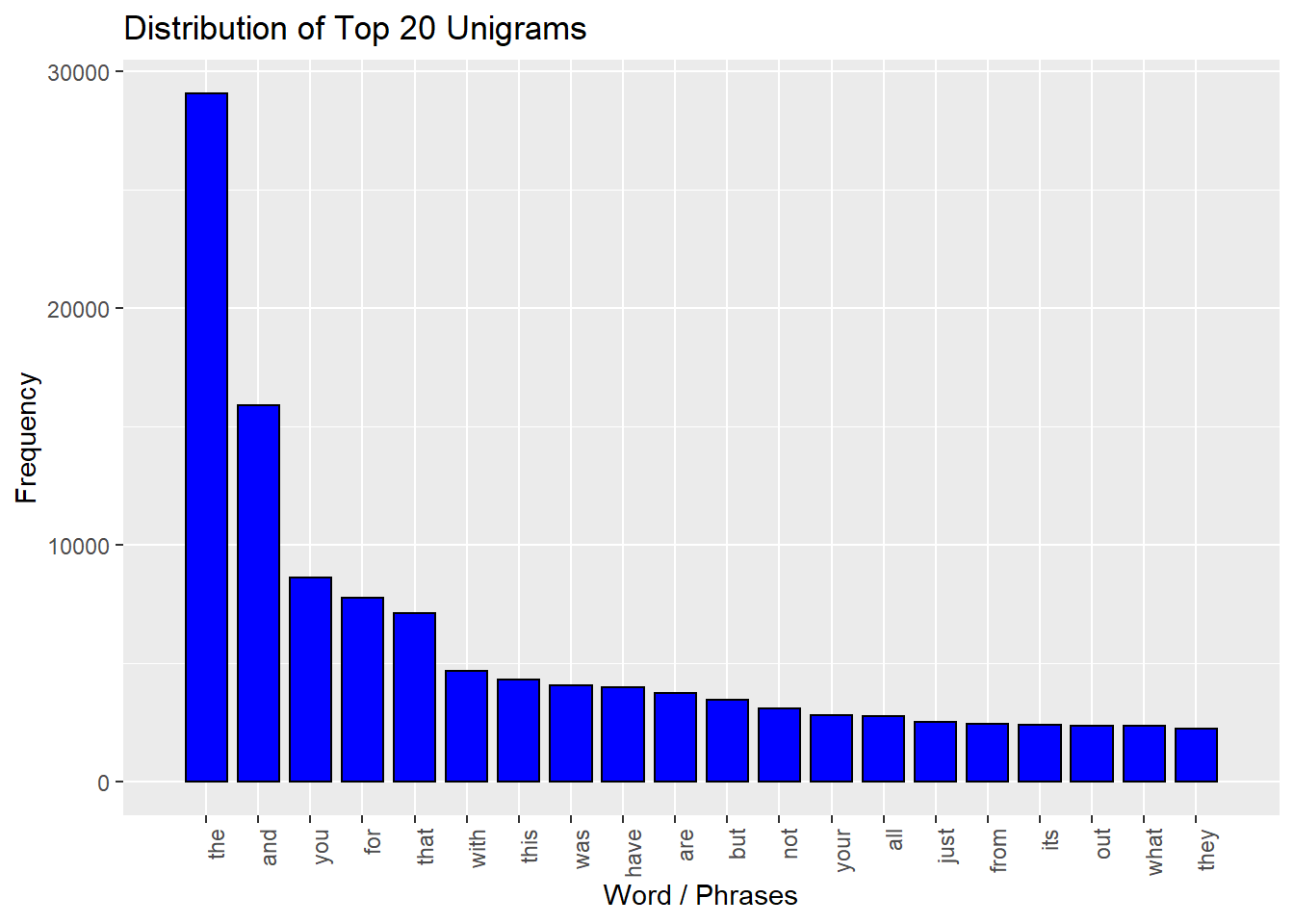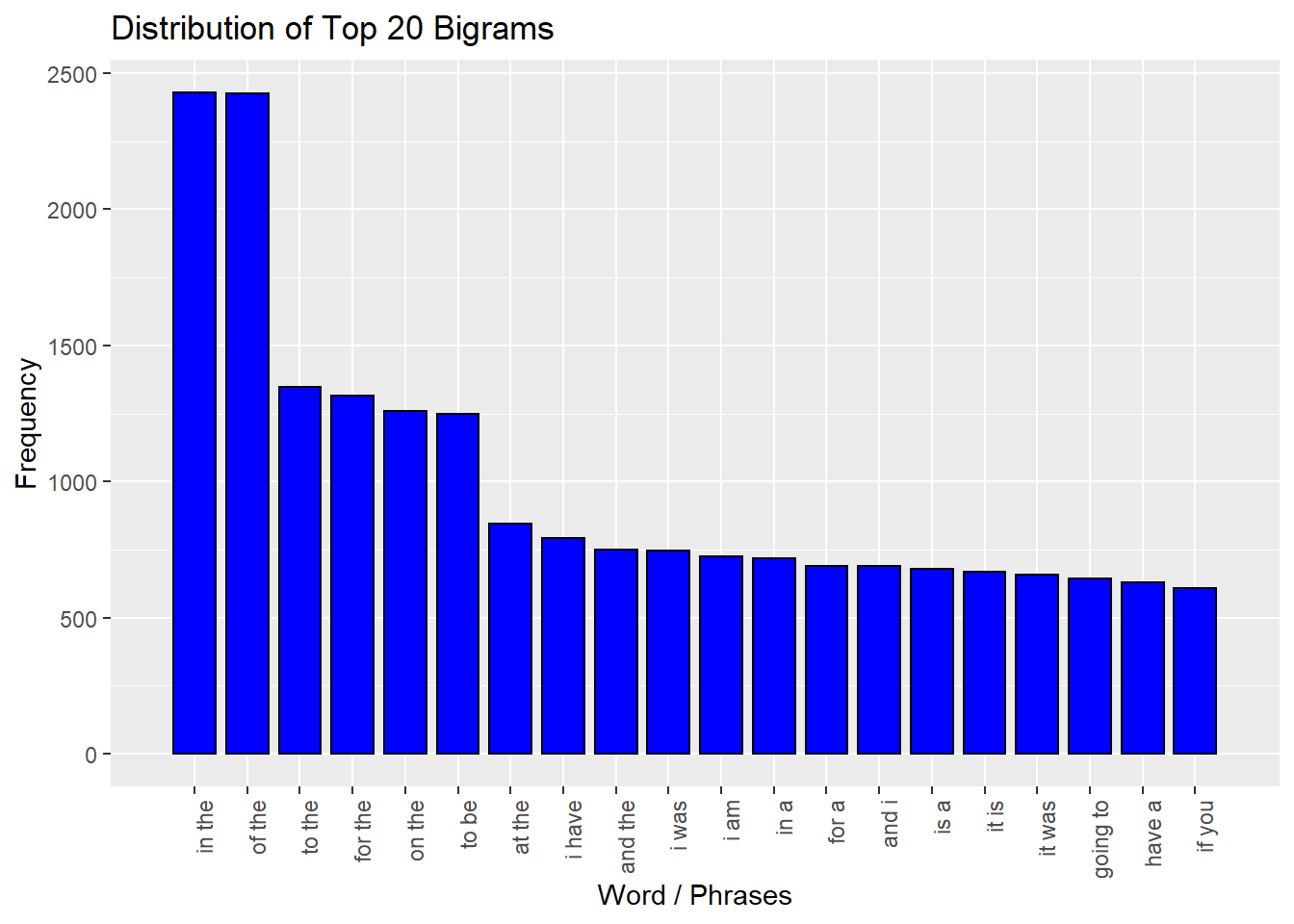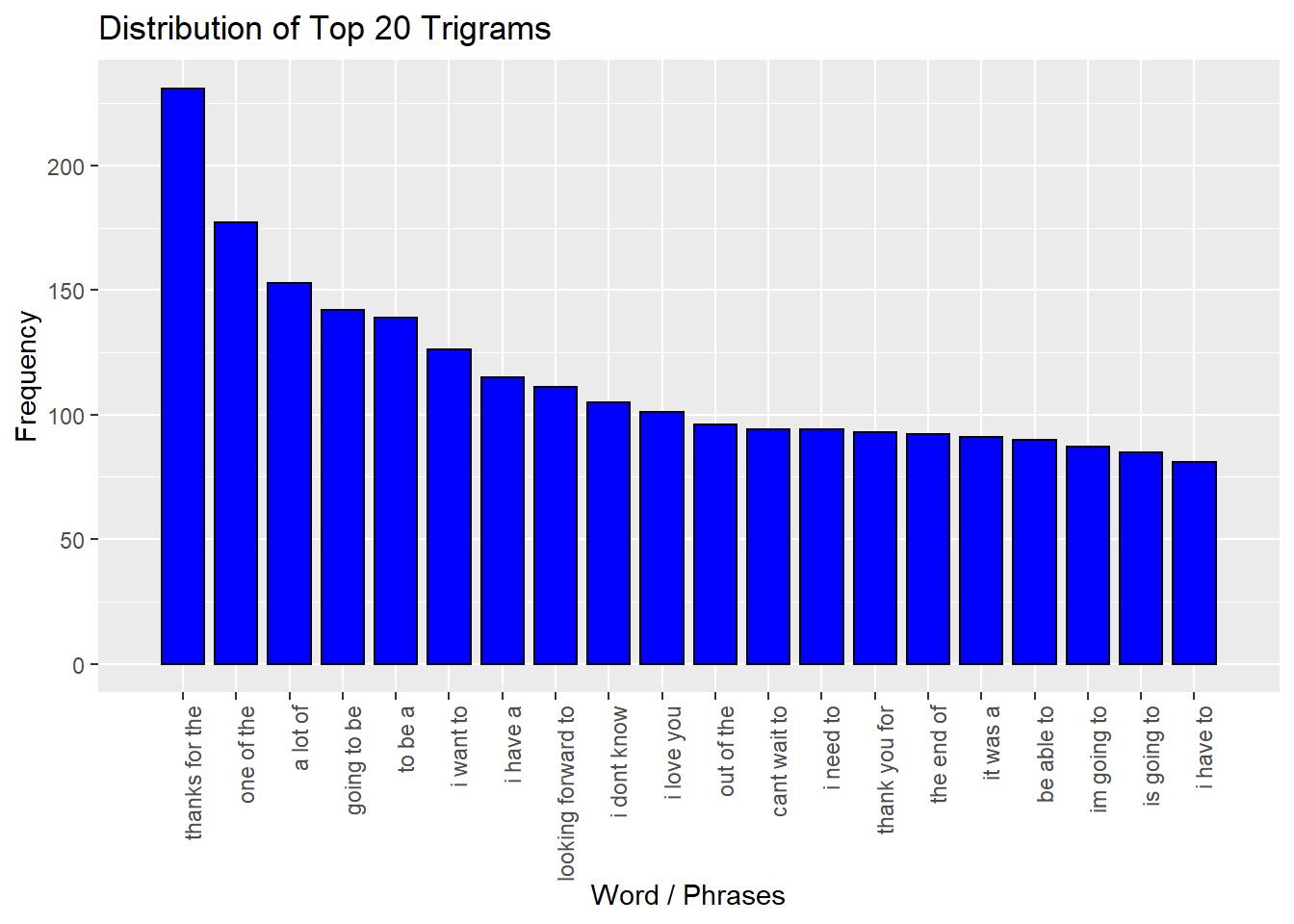## Overview

This is a Milestone Report to explain my exploratory analysis and goals for my app and algorithm that are to be used for my Coursera Data Science Capstone Project. The goal of this project is to build a predictive text mining application for predicting the relationship between words.

1. Building a Basic n-gram Model: - Using the exploratory analysis I performed, build a basic n-gram model for predicting the next word based on the previous 1, 2, or 3 words.

2. Building a Model to Handle Unseen n-grams: - In some cases, people will want to type a combination of words that does not appear in the corpora. - Build a model to handle cases where a particular n-gram is not observed.

Our n-gram model will be trained with a corpus compiled from 3 sources: news, blogs and twitter. We will use Natural Language Processing (NLP) R packages such as ‘tm’ and ‘RWeka’ to tokenize n-grams as our first step towards building a predictive text mining application.

# Loading Libraries
library(RWeka)
library(dplyr)
##
## Attaching package: 'dplyr'
## The following objects are masked from 'package:stats':
##
##     filter, lag
## The following objects are masked from 'package:base':
##
##     intersect, setdiff, setequal, union
library(stringi)
library(tm)
## Loading required package: NLP
library(ggplot2)
##
## Attaching package: 'ggplot2'
## The following object is masked from 'package:NLP':
##
##     annotate
# Loading Data
news <- readLines("C:/Users/chenl/Desktop/Coursera-SwiftKey/final/en_US/en_US.news.txt", encoding = "UTF-8", skipNul = TRUE, warn=FALSE)
blogs <- readLines("C:/Users/chenl/Desktop/Coursera-SwiftKey/final/en_US/en_US.blogs.txt", encoding = "UTF-8", skipNul = TRUE, warn=FALSE)
twitter <- readLines("C:/Users/chenl/Desktop/Coursera-SwiftKey/final/en_US/en_US.twitter.txt", encoding = "UTF-8", skipNul = TRUE, warn=FALSE)

## Summary Statistics

These are the basic summaries of the 3 sources: news, blogs and twitter. They include word counts, line counts, character counts, number of words per line (min, mean and max) and a basic data table.

# Setting Up Statistics for Number of Words Per Line (WPL)
rownames(WPL)=c('WPL_Min','WPL_Mean','WPL_Max')

# Setting up Data Frame for Summary Statistics

# Illustrating the Headers of Basic Data Table for Summary Statistics
head(stats)
##   Dataset   Lines     Chars    Words WPL_Min WPL_Mean WPL_Max
## 1    news   77259  15639408  2651432       1 34.61779    1123
## 2   blogs  899288 206824382 37570839       0 41.75107    6726
## 3 twitter 2360148 162096241 30451170       1 12.75065      47

As shown in the data table above, blogs have the most number of words per line while tweets have the least number of words per line. This is to be expected due to the character limit in Twitter.

## Building the Corpus

# Cleaning Data by Removing Non-English Characters
news <- iconv(news, "latin1", "ASCII", sub="")
blogs <- iconv(blogs, "latin1", "ASCII", sub="")

# Sampling 1% of Data from Each of the 3 Sources
set.seed(519)
sample_data <- c(sample(news, length(news) * 0.01),
sample(blogs, length(blogs) * 0.01),

# Using the 'tm' Package
corpus <- VCorpus(VectorSource(sample_data))

# Converting Corpus to Plain Text
corpus <- tm_map(corpus, tolower)
corpus <- tm_map(corpus, removePunctuation)
corpus <- tm_map(corpus, removeNumbers)
corpus <- tm_map(corpus, stripWhitespace)
corpus <- tm_map(corpus, PlainTextDocument)

## Tokenization and Calculation of Frequencies for N-Grams

# Using the 'RWeka' Package for Tokenization
uni_tokenizer <- function(x) NGramTokenizer(x, Weka_control(min = 1, max = 1))
bi_tokenizer <- function(x) NGramTokenizer(x, Weka_control(min = 2, max = 2))
tri_tokenizer <- function(x) NGramTokenizer(x, Weka_control(min = 3, max = 3))

# Constructing Matrices of Unigrams, Bigrams and Trigrams
uni_matrix <- TermDocumentMatrix(corpus, control = list(tokenize = uni_tokenizer))
bi_matrix <- TermDocumentMatrix(corpus, control = list(tokenize = bi_tokenizer))
tri_matrix <- TermDocumentMatrix(corpus, control = list(tokenize = tri_tokenizer))

# Calculating Frequencies of N-Grams
uni_corpus <- findFreqTerms(uni_matrix,lowfreq = 50)
bi_corpus <- findFreqTerms(bi_matrix,lowfreq=50)
tri_corpus <- findFreqTerms(tri_matrix,lowfreq=50)

# Constructing Data Frames of Frequencies
uni_corpus_freq <- rowSums(as.matrix(uni_matrix[uni_corpus,]))
uni_corpus_freq <- data.frame(word=names(uni_corpus_freq), frequency=uni_corpus_freq)
bi_corpus_freq <- rowSums(as.matrix(bi_matrix[bi_corpus,]))
bi_corpus_freq <- data.frame(word=names(bi_corpus_freq), frequency=bi_corpus_freq)
tri_corpus_freq <- rowSums(as.matrix(tri_matrix[tri_corpus,]))
tri_corpus_freq <- data.frame(word=names(tri_corpus_freq), frequency=tri_corpus_freq)
head(uni_corpus_freq)
##                  word frequency
## able             able       213
## above           above       106
## absolutely absolutely        67
## according   according        93
## account       account        80
head (bi_corpus_freq)
##              word frequency
## a big       a big       110
## a bit       a bit       177
## a book     a book        50
## a couple a couple       121
## a day       a day        76
head(tri_corpus_freq)
##                    word frequency
## a bit of       a bit of        51
## a couple of a couple of        72
## a lot of       a lot of       153
## all of the   all of the        73
## as well as   as well as        79
## at the end   at the end        54

## Histograms of N-Grams

We will plot histograms of the top 20 most frequent words and phrases in the 3 N-Grams.

# Plotting Histograms of N-Grams
plot_n_grams <- function(data, title, word) {
df2 <- data[order(-data$frequency),][1:word,] ggplot(df2, aes(factor(x = seq(1:20)), y = frequency)) + geom_bar(stat = "identity", fill = "blue", colour = "black", width = 0.80) + coord_cartesian(xlim = c(0, word+1)) + labs(title = title) + xlab("Word / Phrases") + ylab("Frequency") + scale_x_discrete(breaks = seq(1, word, by = 1), labels = df2$word[1:word]) +
theme(axis.text.x = element_text(angle = 90, hjust = 1))}

# Plotting Distribution of Top 20 Unigrams
plot_n_grams(uni_corpus_freq,"Distribution of Top 20 Unigrams",20)# Plotting Distribution of Top 20 Bigrams
plot_n_grams(bi_corpus_freq,"Distribution of Top 20 Bigrams",20)# Plotting Distribution of Top 20 Trigrams
plot_n_grams(tri_corpus_freq,"Distribution of Top 20 Trigrams",20)## Summary

This report explains our exploratory analysis and goals for our app and algorithm. Our plan is to build a prediction algorithm that uses an n-gram model to lookup the frequencies of words or phrases. We will employ our prediction algorithm in a Shiny app. The app will suggest the most likely word that comes after a word or phrase has been indicated in the app.